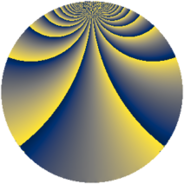# Properties

 Label 912.2.uLevel $912$ Weight $2$ Character orbit 912.u Rep. character $\chi_{912}(229,\cdot)$ Character field $\Q(\zeta_{4})$ Dimension $144$ Newform subspaces $2$ Sturm bound $320$ Trace bound $20$

# Related objects

## Defining parameters

 Level: $$N$$ $$=$$ $$912 = 2^{4} \cdot 3 \cdot 19$$ Weight: $$k$$ $$=$$ $$2$$ Character orbit: $$[\chi]$$ $$=$$ 912.u (of order $$4$$ and degree $$2$$) Character conductor: $$\operatorname{cond}(\chi)$$ $$=$$ $$16$$ Character field: $$\Q(i)$$ Newform subspaces: $$2$$ Sturm bound: $$320$$ Trace bound: $$20$$ Distinguishing $$T_p$$: $$5$$

## Dimensions

The following table gives the dimensions of various subspaces of $$M_{2}(912, [\chi])$$.

Total New Old
Modular forms 328 144 184
Cusp forms 312 144 168
Eisenstein series 16 0 16

## Trace form

 $$144q + 8q^{4} + O(q^{10})$$ $$144q + 8q^{4} + 16q^{11} - 16q^{12} - 24q^{14} - 8q^{16} - 8q^{18} - 32q^{20} + 24q^{28} + 32q^{29} + 16q^{30} + 32q^{34} + 32q^{37} + 8q^{40} + 40q^{42} + 16q^{43} + 24q^{44} + 32q^{48} - 144q^{49} + 56q^{50} + 64q^{52} - 32q^{53} - 56q^{56} - 24q^{58} - 64q^{59} + 48q^{62} - 16q^{63} + 56q^{64} + 32q^{65} - 16q^{67} - 24q^{68} - 40q^{70} + 8q^{72} - 144q^{74} - 32q^{75} - 32q^{77} - 24q^{78} + 88q^{80} - 144q^{81} - 40q^{82} + 48q^{84} + 96q^{86} - 64q^{88} + 16q^{90} + 16q^{91} + 8q^{92} + 24q^{94} - 40q^{96} + 160q^{98} + 16q^{99} + O(q^{100})$$

## Decomposition of $$S_{2}^{\mathrm{new}}(912, [\chi])$$ into newform subspaces

Label Dim. $$A$$ Field CM Traces $q$-expansion
$$a_2$$ $$a_3$$ $$a_5$$ $$a_7$$
912.2.u.a $$72$$ $$7.282$$ None $$0$$ $$0$$ $$0$$ $$0$$
912.2.u.b $$72$$ $$7.282$$ None $$0$$ $$0$$ $$0$$ $$0$$

## Decomposition of $$S_{2}^{\mathrm{old}}(912, [\chi])$$ into lower level spaces

$$S_{2}^{\mathrm{old}}(912, [\chi]) \cong$$ $$S_{2}^{\mathrm{new}}(16, [\chi])$$$$^{\oplus 4}$$$$\oplus$$$$S_{2}^{\mathrm{new}}(48, [\chi])$$$$^{\oplus 2}$$$$\oplus$$$$S_{2}^{\mathrm{new}}(304, [\chi])$$$$^{\oplus 2}$$